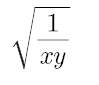# Which function is appropriateI want to express such a function form, in which both x and y are convex, but cvx does not accept this form, after I checked some functions about cvx, I found that there is no more suitable function, can someone help me take a look at this Which function is better in functional form?

By introducing a new variable z, your problem will become how to express \sqrt {\frac{1}{{xy}}} \le z. Then do \log \left( {\sqrt {\frac{1}{{xy}}} } \right) \le \log z, simplify it, then it can be expressed using log().

or Use inv_pos(z)<=geo_mean([x,y])

I don’t know if you mean that

，In this case，-rel_entr function also is not available.